# 活动:沙漠步行 2

## 摔机！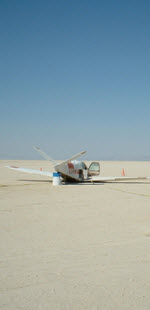如果你还不认识翠翠，你应该先去做 沙漠步行 这个活动。

• 从飞机拿 一瓶水和一个罗盘，
• 然后向北走 1 km， 转方向， 向东走 2 km，再向南 3 km，向西 4 km，向北 5 km，向东 6 km，……像这样：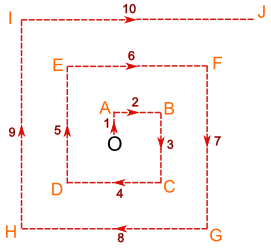• 先从北方计量
• 顺时针方向计量
• 计量方位到三位（或多过三位，如果有小数点）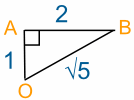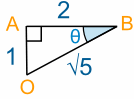tan(θ) = opposite/adjacent （对边/邻边）= 1/2 = 0.5

θ = 26.6°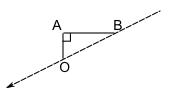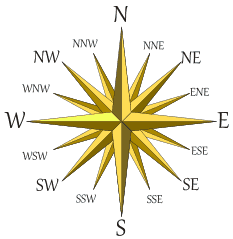这角度是在南与西之间，较近西。 就叫西南偏西吧。 可是这不太准确。翠翠回不到飞机了！ 在这情况下可能不重要，因为 B 离飞机不远，她可能可以看到飞机。 但是在其他地方就需要准确很多了.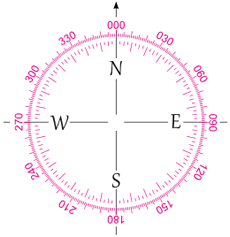## 什么是三位方位角？

• 从北开始计量
• 顺时针方向计量
• 用三位（或更多，如果有小数）有效数字来表达方位

### 例子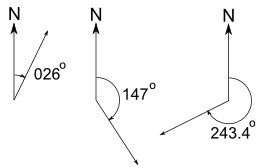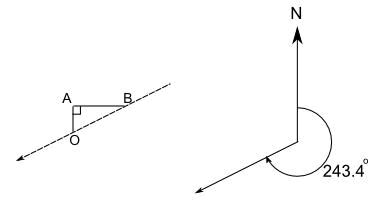## 轮到你了

（注意:距离是在 沙漠步行里计算的）。

 点 总共走的 距离 距离 O 的 直线距离 回到 O 到 三位方位角 O 0 0 不适用 A 1 1 180° B 3 √5 243.4° C 6 D E

## 用极坐标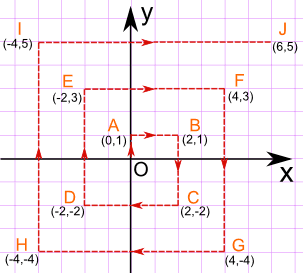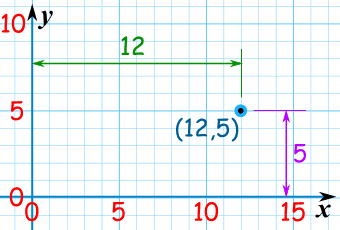r = √( x2 + y2 )

θ = tan-1 ( y / x )

r = √( x2 + y2 )= √( 22 + 12 )= √( 4 + 1 )= √5

θ = tan-1 ( y / x ) = tan-1 ( 1/2 ) = 26.6°

B 点的极坐标是 (√5, 26.6°)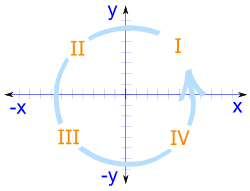基于点所在的 象限 ，有一条简单的规则： 在象限 I、 I 和 III （B, F, J, E, I, D 和 H 点）， 把 270° 减去角度 象限 IV （C 和 G 点）， 把 630° 减去角度 （是 630°，不是 360°）

B 点 （在象限 I）， θ = 26.6°，三位方位角是 270° - 26.6° = 243.4°

### I 点, x= -4 和 y = 5，所以：

r = √( x2 + y2 )= √( (-4)2 + 52 )= √( 16 + 25 )= √41

θ = tan-1 ( y / x ) = tan-1 ( 5/-4 ) = tan-1 (-1.25) = 128.7°

I 是在象限 II，所以三位方位角是 270° - 128.7° = 141.3°

 点 r 的值 θ 的值 极坐标 回到 O 的 三位方位角 O 0 0° (0, 0°) 不适用 A 1 90° (1, 90°) 180° B √5 26.6° (√5, 26.6°) 243.4° C D E F G H I √41 128.7° (√41, 128.7°) 141.3° J